How to Use Nested If Statement in Excel VBA?

• Last Updated : 19 Jul, 2021

VBA in Excel stands for Visual Basic for Applications which is Microsoft’s programming language. To optimize the performance and reduce the time in Excel we need Macros and VBA is the tool used in the backend.

In this article, we are going to use how to use the Nested If statement in Excel VBA.

Implementation :

In the Microsoft Excel tabs, select the Developer Tab. Initially, the Developer Tab may not be available.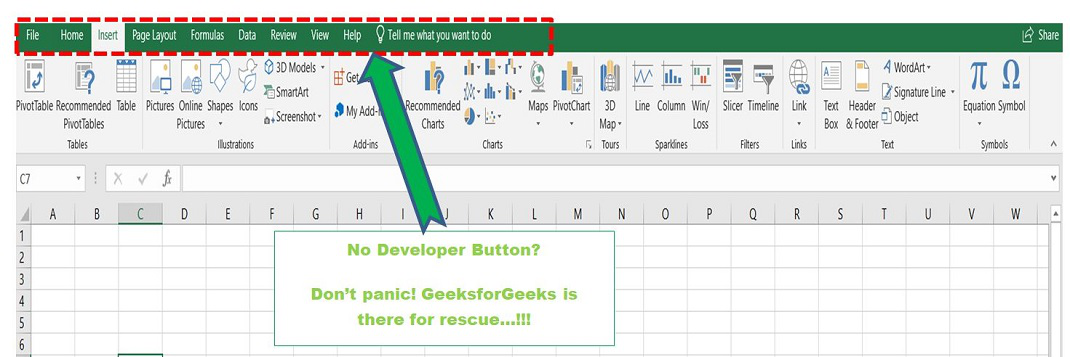The Developer Tab can be enabled easily by a two-step process :

• Right-click on any of the existing tabs at the top of the Excel window.
• Now select Customize the Ribbon from the pop-down menu.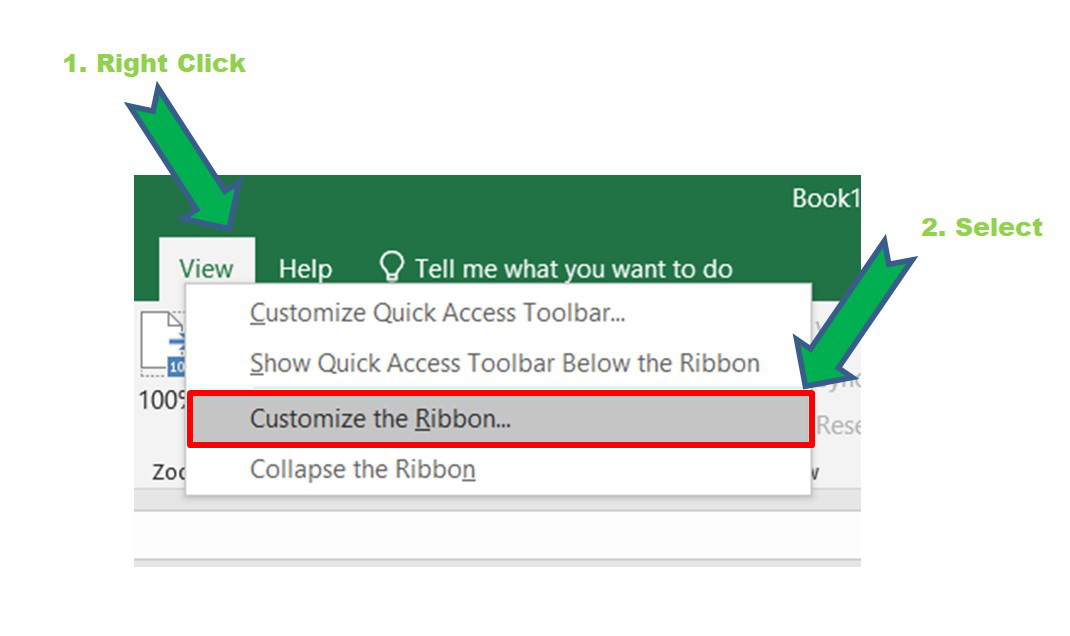• In the Excel Options Box, check the box Developer to enable it and click on OK.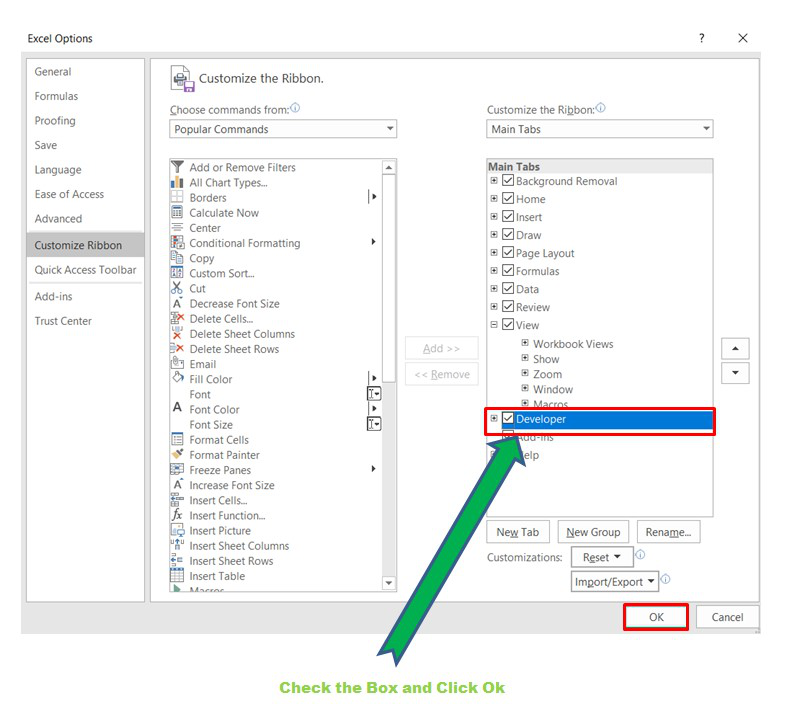• Now, the Developer Tab is visible.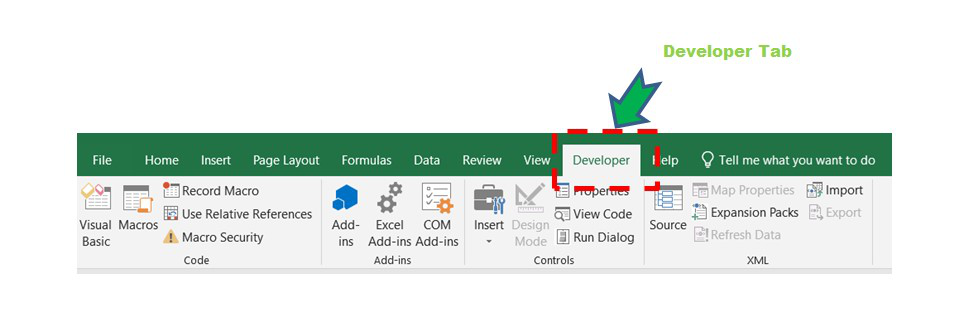• Now click on the Visual Basic option in the Developer tab and make a new module to write the program using the Select Case statement.
Developer  -> Visual Basic -> Tools -> Macros
• Now create a Macro and give any suitable name.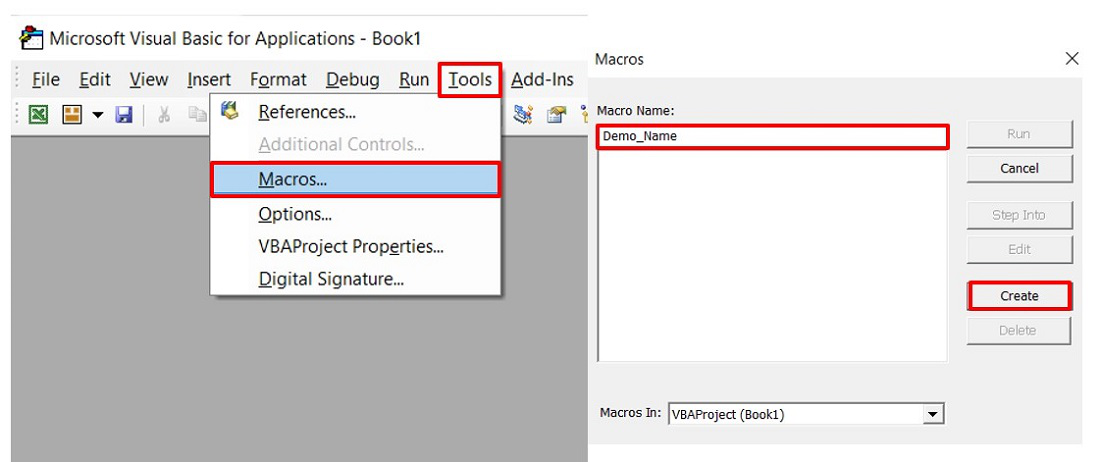• This will open the Editor window where can write the code.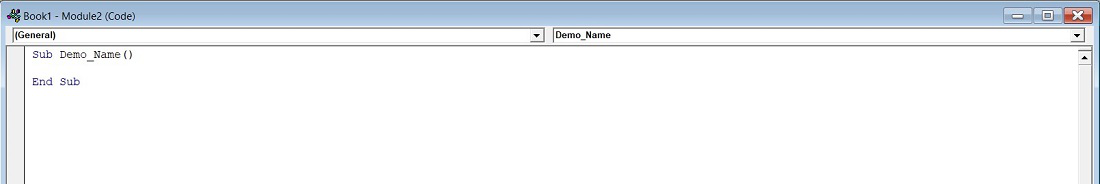The syntax for the If statement in Excel is :

If condition/expression Then
Code Block for True
Else
Code Block for False
End If

Nested IF:

The structure of Nested If statement in Excel is :

If condition/expression Then
Code Block 1
Else If condition/expression Then
Code Block 2
Else If condition/expression Then
Code Block 3
Else
Code Block 4
Else
Code Block 5
End If

Some important keywords used in Excel are as follows :

1. InputBox : To take input from the user.
2. MsgBox : To display output to the user.

Example: Consider a grading system where grading is based on the marks obtained in the exam. For example, If a student obtained 95 marks the grade obtained by the student is S grade and so on.

Code :

'Declaring the variable marks
Dim marks As Integer
If marks >= 90 Then
Else
If marks >= 80 Then
Else
If marks >= 70 Then
Else
If marks >= 60 Then
Else
If marks >= 50 Then
Else
If marks >= 40 Then
Else
MsgBox "You have failed in the exam"
End If
End If
End If
End If
End If
End If
End Sub

Result :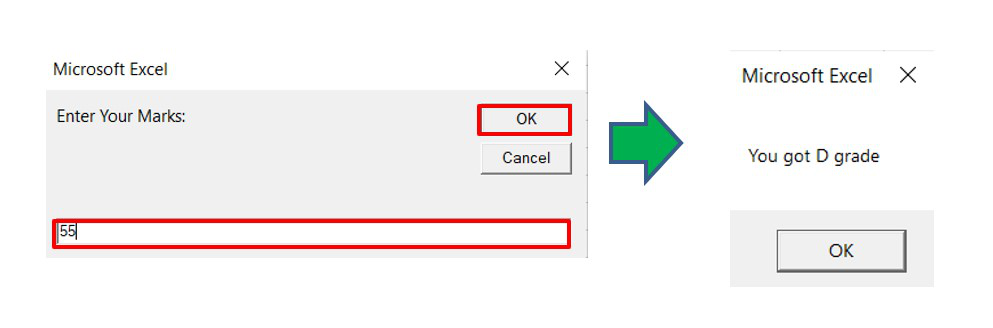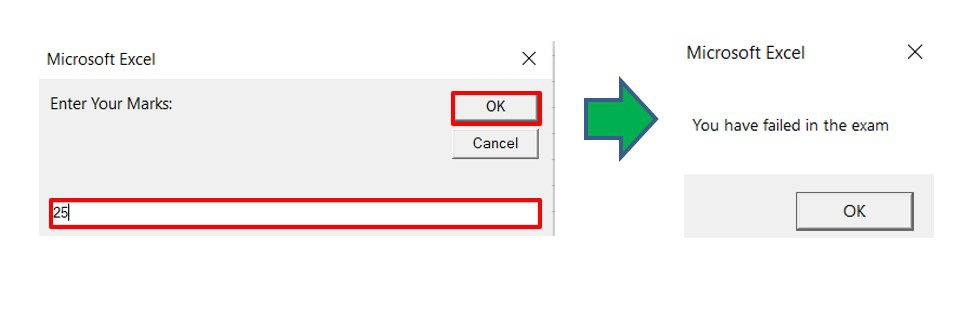Attention reader! Don’t stop learning now. If you are an Excel beginner (or an intermediate) and want to learn Excel, Geeksforgeeks brings the perfect course for you to start, Diving Into Excel

My Personal Notes arrow_drop_up